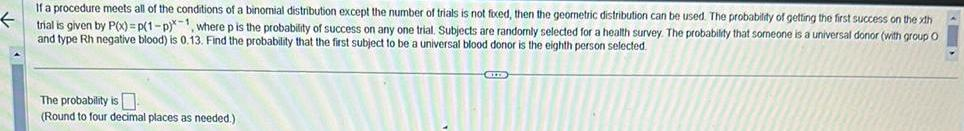Question:

# If a procedure meets all of the conditions of a binomial

Last updated: 3/18/2023If a procedure meets all of the conditions of a binomial distribution except the number of trials is not fixed then the geometric distribution can be used The probability of getting the first success on the xth trial is given by P x P 1 p 1 where p is the probability of success on any one trial Subjects are randomly selected for a health survey The probability that someone is a universal donor with group O and type Rh negative blood is 0 13 Find the probability that the first subject to be a universal blood donor is the eighth person selected The probability is Round to four decimal places as needed